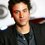# Wau - Whats your thought on it?

Main post link -> http://www.youtube.com/watch?v=GFLkou8NvJo

Have a nice look at the video and comment on what you think of it? A video by Vi Hart (a Mathemusician) Does that number really exist? Or is it hypothetical? What are your views on it? From me, Its just Wow.Note by Nishanth Hegde
6 years, 2 months ago

MarkdownAppears as
*italics* or _italics_ italics
**bold** or __bold__ bold
- bulleted- list
• bulleted
• list
1. numbered2. list
1. numbered
2. list
Note: you must add a full line of space before and after lists for them to show up correctly
paragraph 1paragraph 2

paragraph 1

paragraph 2

[example link](https://brilliant.org)example link
> This is a quote
This is a quote
    # I indented these lines
# 4 spaces, and now they show
# up as a code block.

print "hello world"
# I indented these lines
# 4 spaces, and now they show
# up as a code block.

print "hello world"
MathAppears as
Remember to wrap math in $ ... $ or $ ... $ to ensure proper formatting.
2 \times 3 $2 \times 3$
2^{34} $2^{34}$
a_{i-1} $a_{i-1}$
\frac{2}{3} $\frac{2}{3}$
\sqrt{2} $\sqrt{2}$
\sum_{i=1}^3 $\sum_{i=1}^3$
\sin \theta $\sin \theta$
\boxed{123} $\boxed{123}$

## Comments

Sort by:

Top Newest

It turns out that wau equals one. That's the reason why many of these sequences work. For example, take the first infinite fraction: $\dfrac{2}{\dfrac3{\dfrac3{\dfrac3{\cdots}+\dfrac1{\cdots}}+\dfrac1{\dfrac3{\cdots}+\dfrac1{\cdots}}}+\dfrac1{\dfrac3{\dfrac3{\cdots}+\dfrac1{\cdots}}+\dfrac1{\dfrac3{\cdots}+\dfrac1{\cdots}}}}$ For simplicity, let $x=\dfrac{1}{\dfrac3{\dfrac3{\dfrac3{\cdots}+\dfrac1{\cdots}}+\dfrac1{\dfrac3{\cdots}+\dfrac1{\cdots}}}+\dfrac1{\dfrac3{\dfrac3{\cdots}+\dfrac1{\cdots}}+\dfrac1{\dfrac3{\cdots}+\dfrac1{\cdots}}}}.$ Then we get $x=\dfrac{1}{3x+1x}=\dfrac1{4x}\implies x^2=\dfrac14\implies x=\dfrac12,$ so $F=2x=1$ as desired. In addition, a lot of the exponentiation infinite series crumble under the definition that $F=1$, since $1^{\text{anything}}=1$.

Furthermore, how legitimate is a number whose name sounds similar to the word "wow"? ;)

- 6 years, 2 months ago

Log in to reply

Yeah. The most straightforward clue in the video that wau is 1 is that she says $e^{2i \pi} = F$ which obviously is one.

- 6 years, 2 months ago

Log in to reply

There were other early clues. the one with 5/6 was easy to evaluate. We get F=5/6+1/6*(F) ->F=1. That was really the moment when I went "wait a minute..."

- 6 years, 2 months ago

Log in to reply

Yeah. But how to justify $F=\frac{x+x^{y}+x^{x^{y}} \ldots }{y+y^{x}+y^{y^{x}} \ldots }$ ?

- 6 years, 2 months ago

Log in to reply

Remember, $x$ and $y$ were side lengths of a rectangle that had its side lengths in the ratio $F$. In other words, $x=y$. Then that fraction collapses.

- 6 years, 2 months ago

Log in to reply

David A. .... VERY BRILLIANT THINKING....GREAT ........

- 6 years, 2 months ago

Log in to reply

I have an issue with her initial definition of "wau". It appears that the continued fraction she starts with, is in fact, not convergent. As she pointed out, the partial values of the partial fraction oscillate between 1/2 and 2; so it does not converge to 1.

It would be like saying 1-1+1-1+1... = x, so 1-x = x and x = 1/2; thus making it seem like the series converges to 1/2. But this is not true under the most "normal" idea of convergence.

- 6 years, 2 months ago

Log in to reply

The number is actually 1

- 6 years, 2 months ago

Log in to reply

ya

- 6 years, 2 months ago

Log in to reply

David A. got the answer

- 6 years, 2 months ago

Log in to reply

'wau'- wow...

- 6 years, 2 months ago

Log in to reply

Yup mate! IT"S REALLY WOW!

- 6 years, 2 months ago

Log in to reply

×

Problem Loading...

Note Loading...

Set Loading...